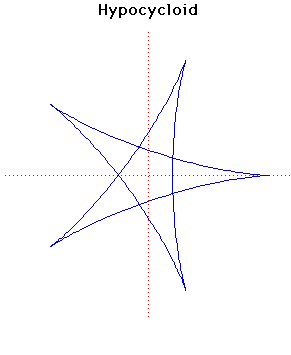# HypocycloidParametric Cartesian equation:
x = (a - b) cos(t) + b cos((a/b - 1)t), y = (a - b) sin(t) - b sin((a/b - 1)t)

Click below to see one of the Associated curves.

Click THIS LINK to experiment interactively with this curve and its associated curves.

There are four curves which are closely related. These are the epicycloid, the epitrochoid, the hypocycloid and the hypotrochoid and they are traced by a point P on a circle of radius b which rolls round a fixed circle of radius a.

For the hypocycloid, an example of which is shown above, the circle of radius b rolls on the inside of the circle of radius a. The point P is on the circumference of the circle of radius b. For the example a = 5 and b = 3.

These curves were studied by Dürer (1525), Desargues (1640), Huygens (1679), Leibniz, Newton (1686), de L'Hôpital (1690), Jacob Bernoulli (1690), la Hire (1694), Johann Bernoulli (1695), Daniel Bernoulli (1725), Euler (1745, 1781).

Special cases are a = 3b when a tricuspoid is obtained and a = 4b when an astroid is obtained.

If a = (n + 1)b where n is an integer, then the length of the epicycloid is 8nb and its area is πb2(n2 - n).

The pedal curve, when the pedal point is the centre, is a rhodonea curve.

The evolute of a hypocycloid is a similar hypocycloid - look at the evolute of the hypocycloid above to see it is a similar hypocycloid but smaller in size.

Other Web site:

JOC/EFR/BS January 1997